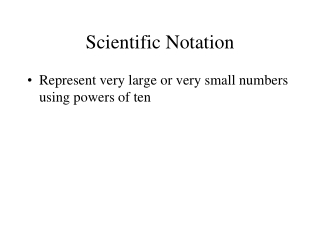DownloadDownload PresentationScientific Notation

Scientific Notation

Download PresentationScientific Notation

- - - - - - - - - - - - - - - - - - - - - - - - - - - E N D - - - - - - - - - - - - - - - - - - - - - - - - - - -
Presentation Transcript

1. Scientific Notation • Represent very large or very small numbers using powers of ten

2. Practice • 5,000 • 0.000265 • 3,510,000,000 • 0.00000071

3. 3.0 x 10-5 • 4.13 x 104 • 9.29 x 102

4. Dimensional Analysis • How do I get from one unit to another? • Set up a conversion factor • Ex: 1 foot = 12 inches or 3 ft = 1 yd

5. Metric Conversions • Kilo Hecta Deca BaseUnit Deci Centi Milli • How many kilometers is 1,500,000 m? • How many cg in 550 dag? • How many kL in 9,256 mL?

6. Convert: • 356 m to km • 2.5 x 10-3 g to mg • 30,050 mL to kL

7. Practice • I want to buy apples. They cost 2.59 per pound. My bag of apples weighs 2.3 pounds. How much will it cost? • 1 pound = \$2.59

8. How many feet are there in the 100 m dash? • How many minutes are there in one week? • How many meters is a 100 yard football field?